Скачать презентацию Chapter 7 Capital Asset Pricing and Arbitrage Pricing

• Количество слайдов: 55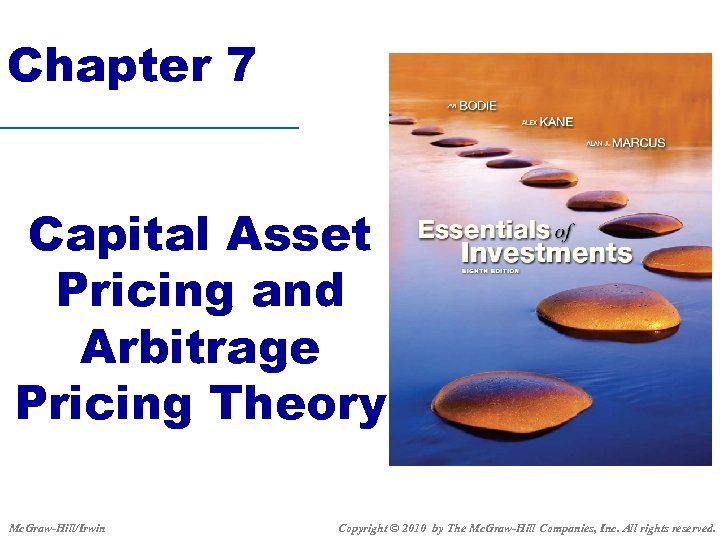Chapter 7 Capital Asset Pricing and Arbitrage Pricing Theory Mc. Graw-Hill/Irwin Copyright © 2010 by The Mc. Graw-Hill Companies, Inc. All rights reserved.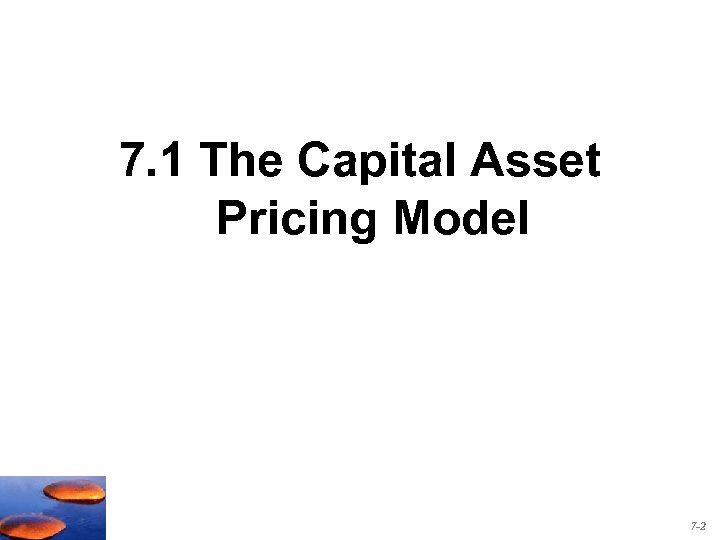7. 1 The Capital Asset Pricing Model 7 -2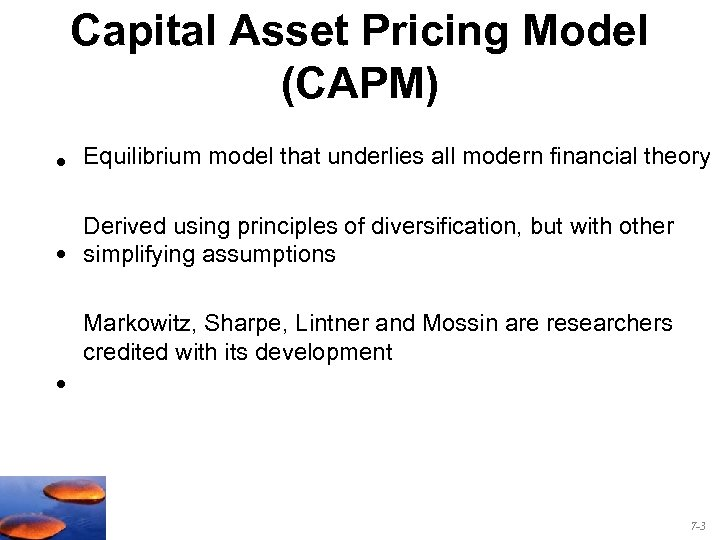Capital Asset Pricing Model (CAPM) • Equilibrium model that underlies all modern financial theory • Derived using principles of diversification, but with other simplifying assumptions Markowitz, Sharpe, Lintner and Mossin are researchers credited with its development • 7 -3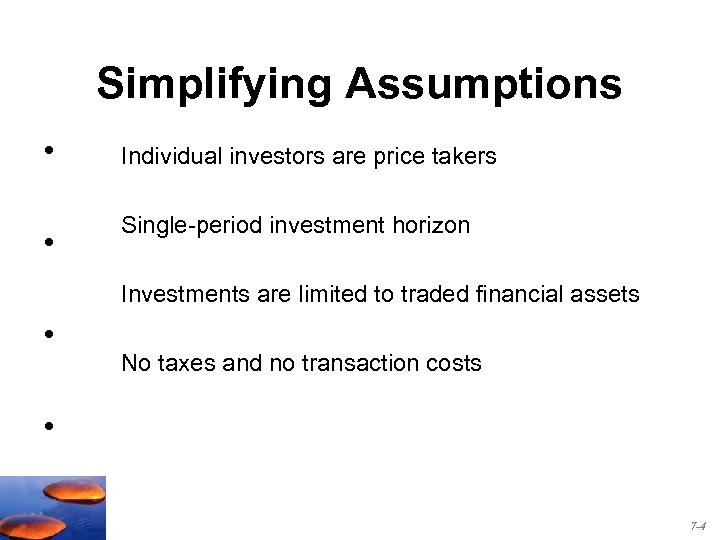Simplifying Assumptions • • Individual investors are price takers Single-period investment horizon Investments are limited to traded financial assets • No taxes and no transaction costs • 7 -4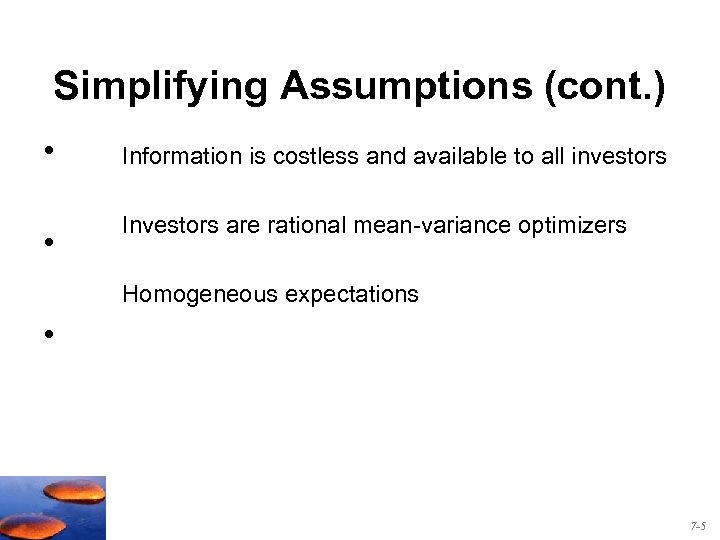Simplifying Assumptions (cont. ) • Information is costless and available to all investors • Investors are rational mean-variance optimizers Homogeneous expectations • 7 -5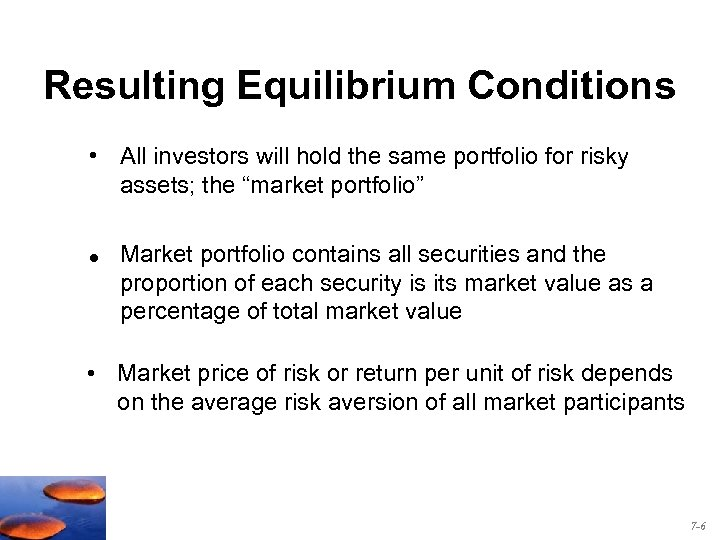Resulting Equilibrium Conditions • All investors will hold the same portfolio for risky assets; the “market portfolio” • Market portfolio contains all securities and the proportion of each security is its market value as a percentage of total market value • Market price of risk or return per unit of risk depends on the average risk aversion of all market participants 7 -6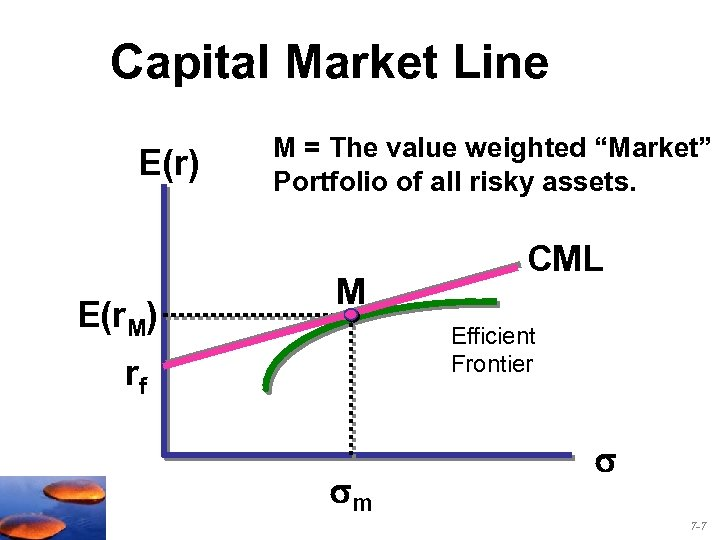Capital Market Line E(r) E(r. M) M = The value weighted “Market” Portfolio of all risky assets. M CML Efficient Frontier rf m 7 -7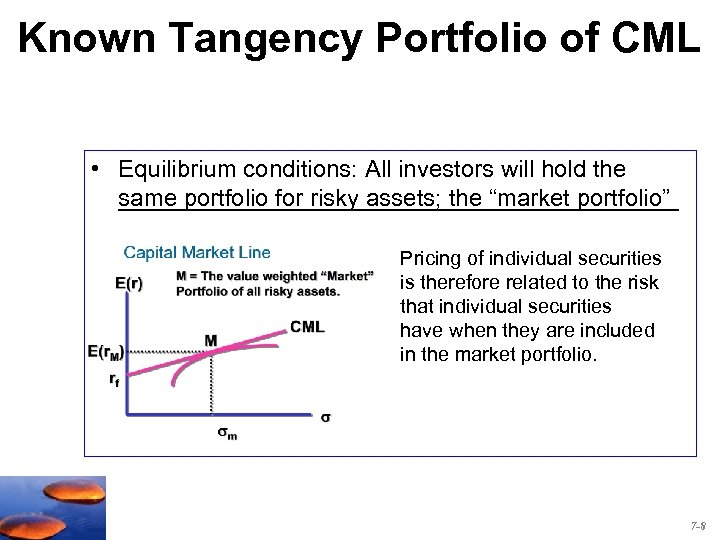Known Tangency Portfolio of CML • Equilibrium conditions: All investors will hold the _____________________ same portfolio for risky assets; the “market portfolio” Pricing of individual securities is therefore related to the risk that individual securities have when they are included in the market portfolio. 7 -8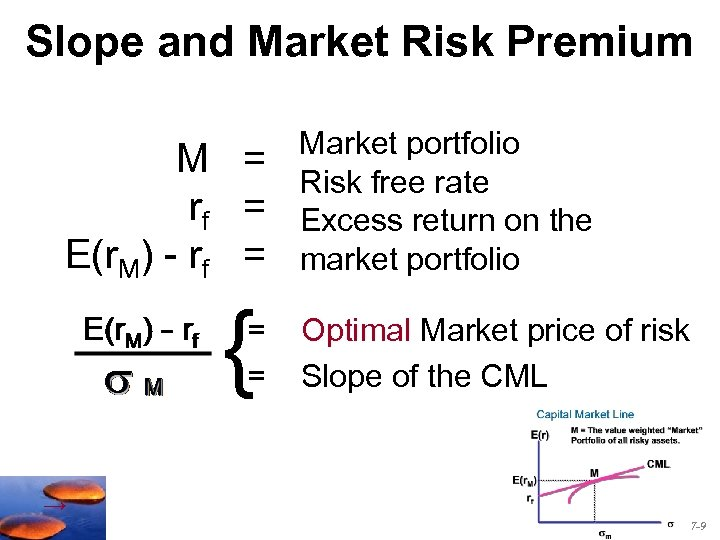Slope and Market Risk Premium M = rf = E(r. M) - rf = { = = Market portfolio Risk free rate Excess return on the market portfolio Optimal Market price of risk Slope of the CML → 7 -9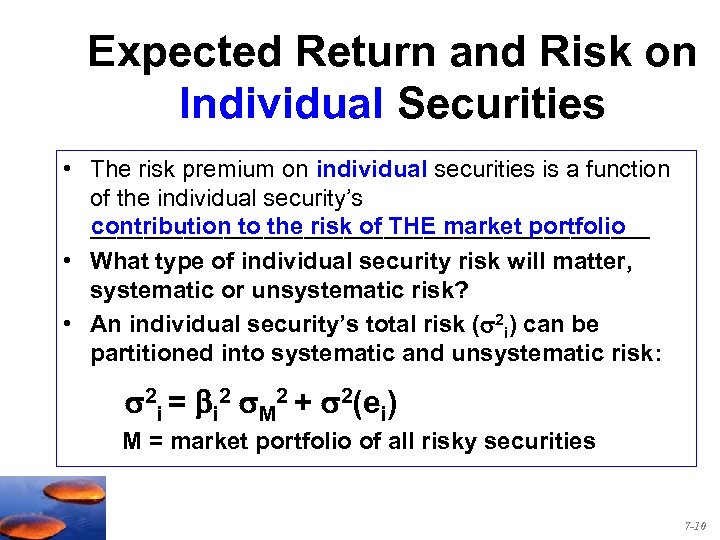Expected Return and Risk on Individual Securities • The risk premium on individual securities is a function of the individual security’s contribution to the risk of THE market portfolio _____________________ • What type of individual security risk will matter, systematic or unsystematic risk? • An individual security’s total risk ( 2 i) can be partitioned into systematic and unsystematic risk: 2 i = bi 2 M 2 + 2(ei) M = market portfolio of all risky securities 7 -10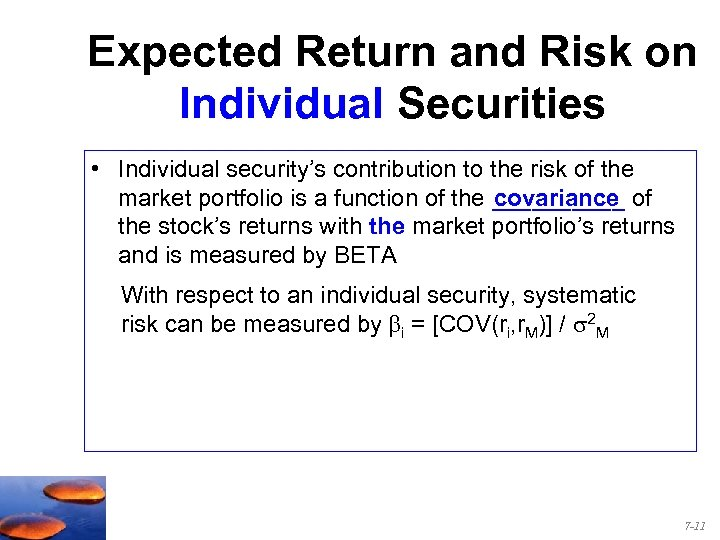Expected Return and Risk on Individual Securities • Individual security’s contribution to the risk of the market portfolio is a function of the _____ of covariance the stock’s returns with the market portfolio’s returns and is measured by BETA With respect to an individual security, systematic risk can be measured by i = [COV(ri, r. M)] / 2 M 7 -11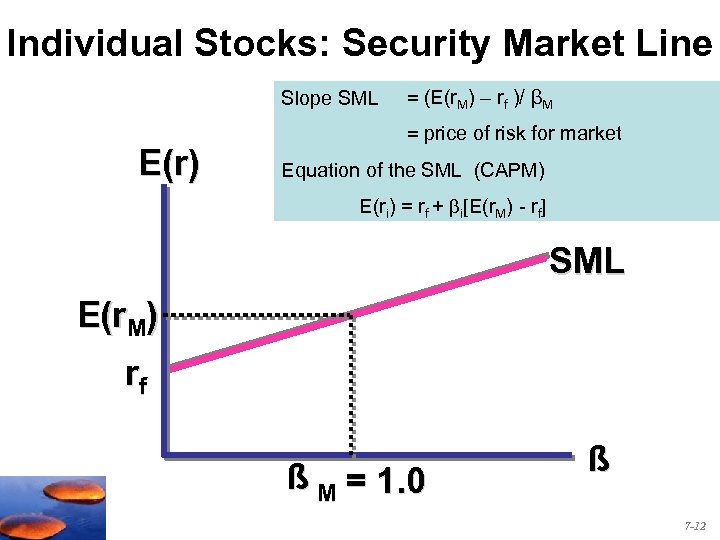Individual Stocks: Security Market Line Slope SML E(r) = (E(r. M) – rf )/ βM = price of risk for market Equation of the SML (CAPM) E(ri) = rf + i[E(r. M) - rf] SML E(r. M) rf ß M = 1. 0 ß 7 -12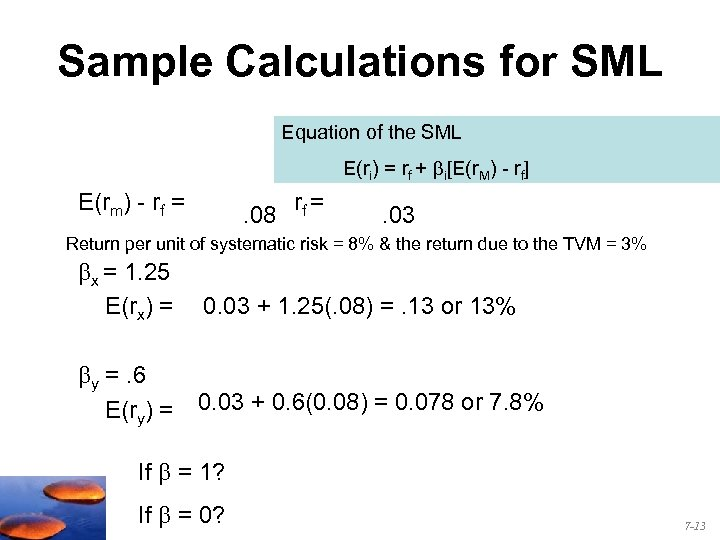Sample Calculations for SML Equation of the SML E(ri) = rf + i[E(r. M) - rf] E(rm) - rf = . 08 rf = . 03 Return per unit of systematic risk = 8% & the return due to the TVM = 3% x = 1. 25 E(rx) = 0. 03 + 1. 25(. 08) =. 13 or 13% y =. 6 E(ry) = 0. 03 + 0. 6(0. 08) = 0. 078 or 7. 8% If = 1? If = 0? 7 -13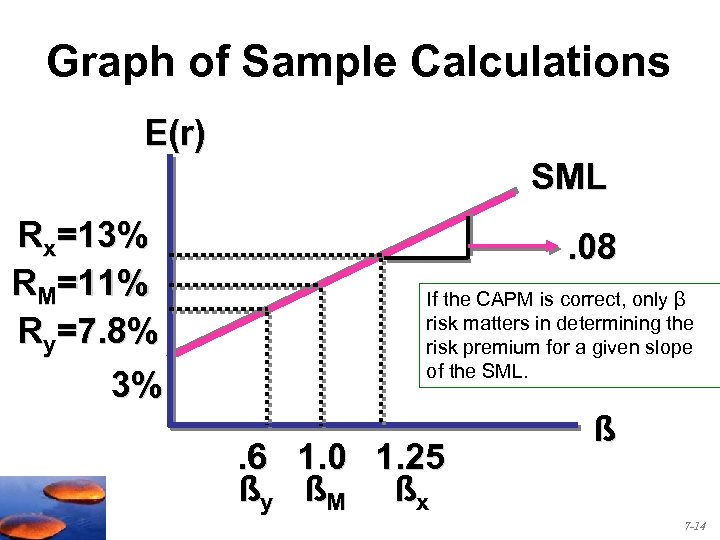Graph of Sample Calculations E(r) SML Rx=13% RM=11% Ry=7. 8% 3% . 08 If the CAPM is correct, only β risk matters in determining the risk premium for a given slope of the SML. . 6 1. 0 1. 25 ßy ßM ßx ß 7 -14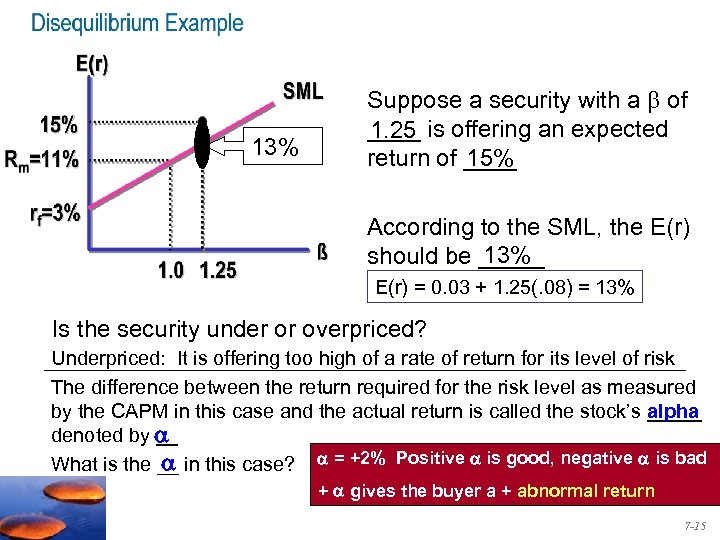13% Suppose a security with a of ____ is offering an expected 1. 25 15% return of ____ According to the SML, the E(r) 13% should be _____ E(r) = 0. 03 + 1. 25(. 08) = 13% Is the security under or overpriced? Underpriced: It is offering too high of a rate of return for its level of risk The difference between the return required for the risk level as measured by the CAPM in this case and the actual return is called the stock’s _____ alpha denoted by __ What is the __ in this case? = +2% Positive is good, negative is bad + gives the buyer a + abnormal return 7 -15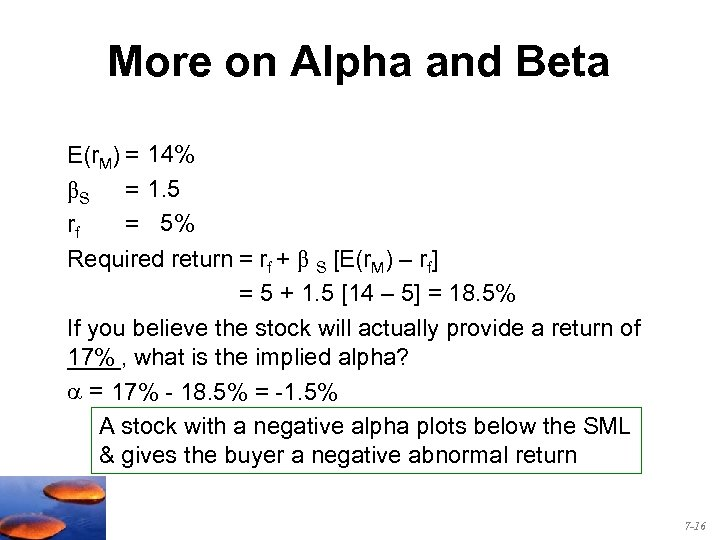More on Alpha and Beta E(r. M) = 14% βS = 1. 5 rf = 5% Required return = rf + β S [E(r. M) – rf] = 5 + 1. 5 [14 – 5] = 18. 5% If you believe the stock will actually provide a return of 17% ____, what is the implied alpha? = 17% - 18. 5% = -1. 5% A stock with a negative alpha plots below the SML & gives the buyer a negative abnormal return 7 -16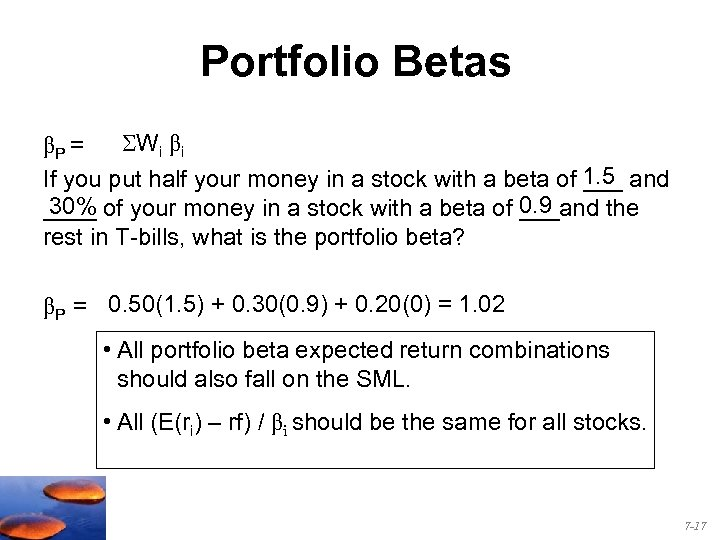Portfolio Betas βP = Wi βi If you put half your money in a stock with a beta of 1. 5 and ___ 0. 9 30% ____ of your money in a stock with a beta of ___and the rest in T-bills, what is the portfolio beta? βP = 0. 50(1. 5) + 0. 30(0. 9) + 0. 20(0) = 1. 02 • All portfolio beta expected return combinations should also fall on the SML. • All (E(ri) – rf) / βi should be the same for all stocks. 7 -17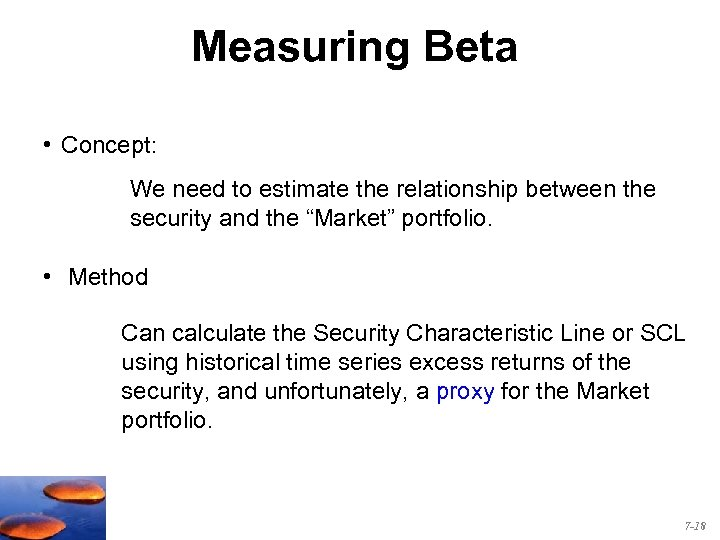Measuring Beta • Concept: We need to estimate the relationship between the security and the “Market” portfolio. • Method Can calculate the Security Characteristic Line or SCL using historical time series excess returns of the security, and unfortunately, a proxy for the Market portfolio. 7 -18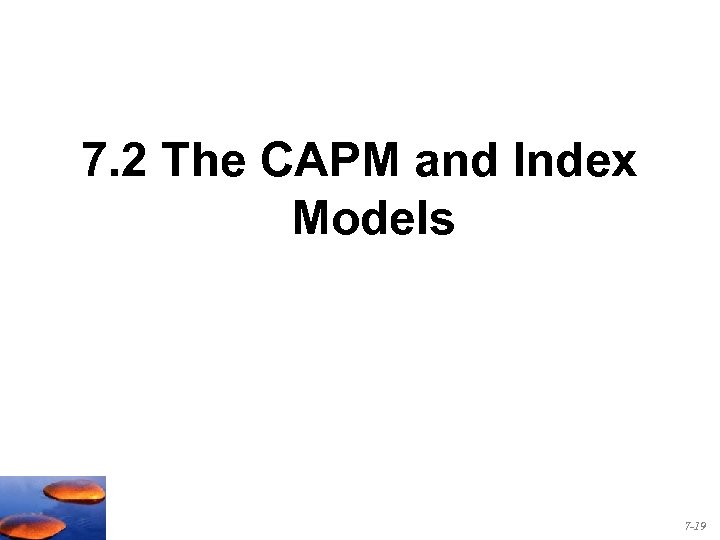7. 2 The CAPM and Index Models 7 -19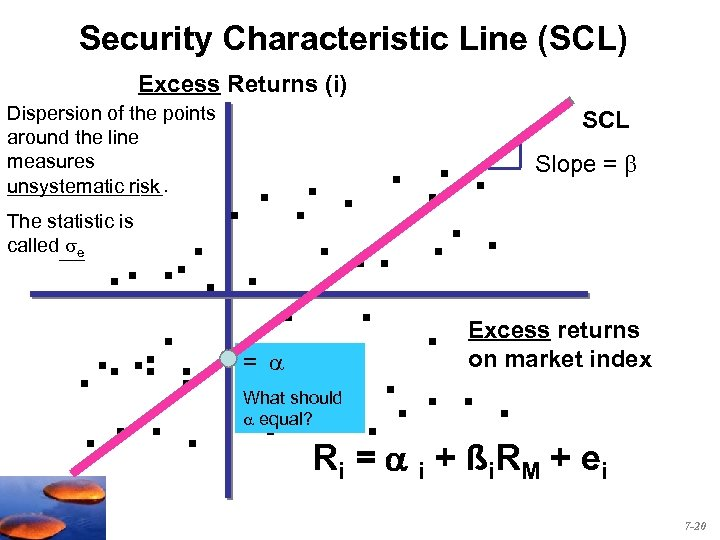Security Characteristic Line (SCL) Excess Returns (i) Dispersion of the points around the line measures _______. unsystematic risk SCL . . Slope = . . . The statistic is. . called . . . Excess returns. . . = . . . on market index. . . . R = + ßR + e e What should equal? i i i M i 7 -20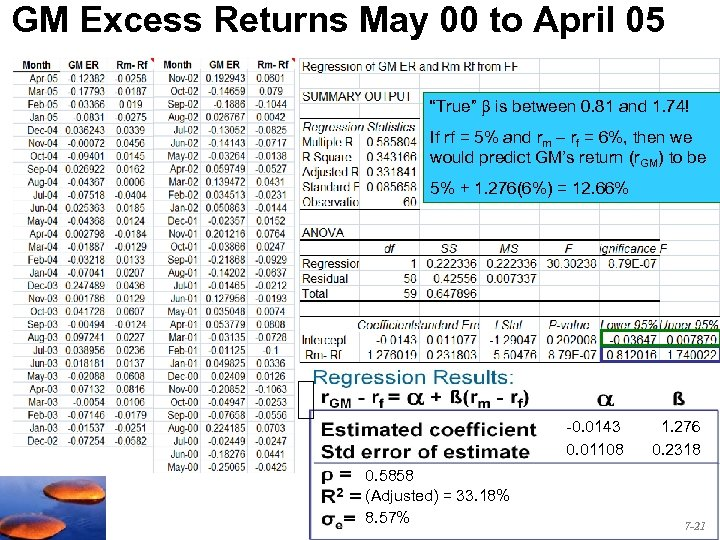GM Excess Returns May 00 to April 05 “True” is between 0. 81 and 1. 74! If rf = 5% and rm – rf = 6%, then we would predict GM’s return (r. GM) to be 5% + 1. 276(6%) = 12. 66% -0. 0143 0. 01108 0. 5858 (Adjusted) = 33. 18% 8. 57% 1. 276 0. 2318 7 -21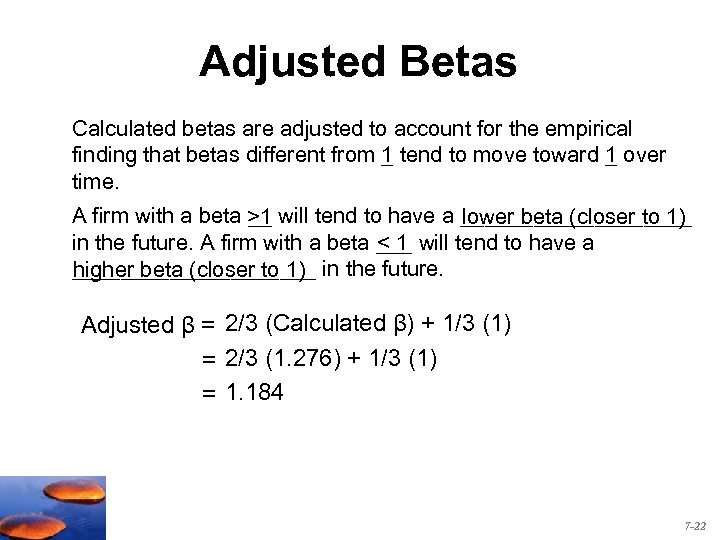Adjusted Betas Calculated betas are adjusted to account for the empirical finding that betas different from 1 tend to move toward 1 over _ _ time. A firm with a beta >1 will tend to have a __________ __ lower beta (closer to 1) in the future. A firm with a beta ___ will tend to have a <1 __________ in the future. higher beta (closer to 1) Adjusted β = 2/3 (Calculated β) + 1/3 (1) = 2/3 (1. 276) + 1/3 (1) = 1. 184 7 -22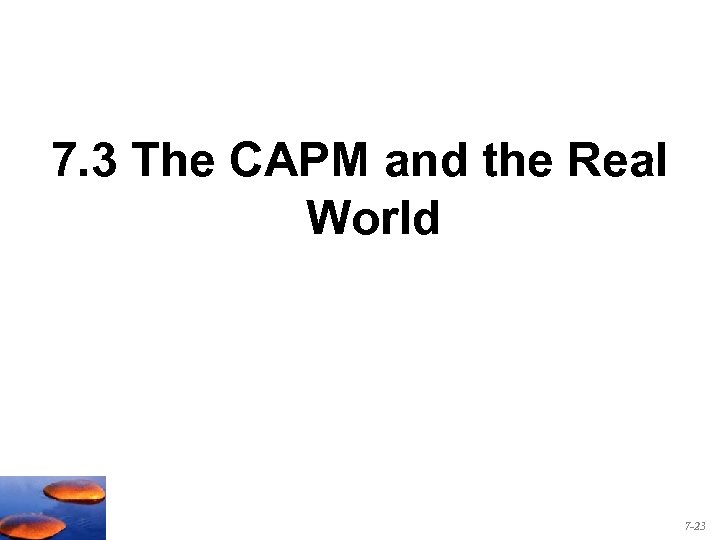7. 3 The CAPM and the Real World 7 -23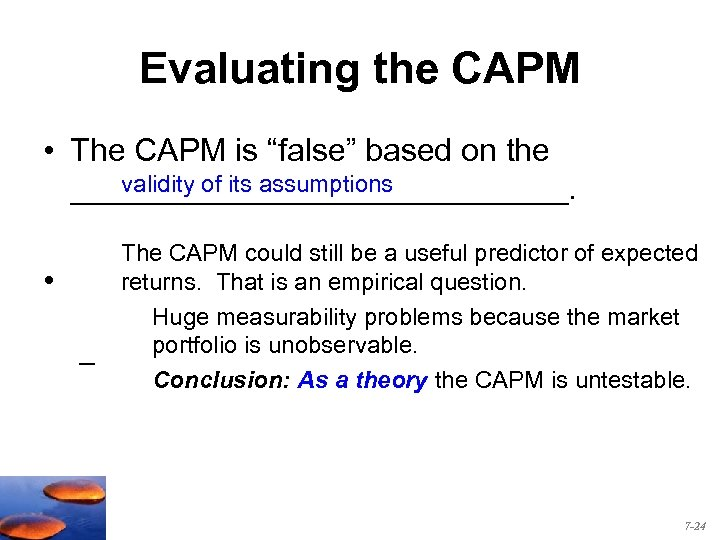Evaluating the CAPM • The CAPM is “false” based on the validity of its assumptions ______________. • – The CAPM could still be a useful predictor of expected returns. That is an empirical question. Huge measurability problems because the market portfolio is unobservable. Conclusion: As a theory the CAPM is untestable. 7 -24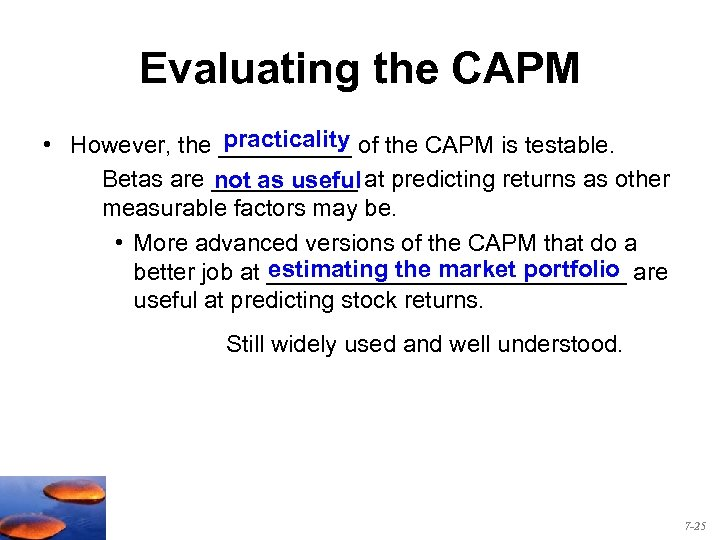Evaluating the CAPM practicality • However, the _____ of the CAPM is testable. Betas are ______ at predicting returns as other not as useful measurable factors may be. • More advanced versions of the CAPM that do a estimating the market portfolio better job at ______________ are useful at predicting stock returns. Still widely used and well understood. 7 -25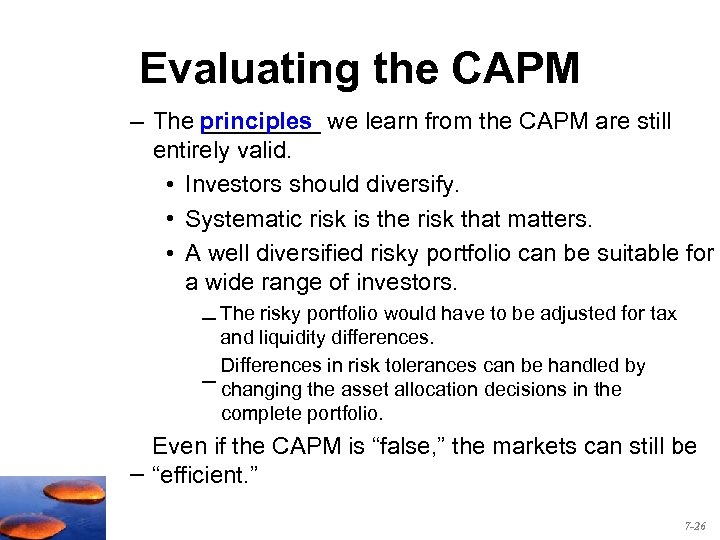Evaluating the CAPM – The principles we learn from the CAPM are still _____ entirely valid. • Investors should diversify. • Systematic risk is the risk that matters. • A well diversified risky portfolio can be suitable for a wide range of investors. – The risky portfolio would have to be adjusted for tax and liquidity differences. Differences in risk tolerances can be handled by – changing the asset allocation decisions in the complete portfolio. Even if the CAPM is “false, ” the markets can still be – “efficient. ” 7 -26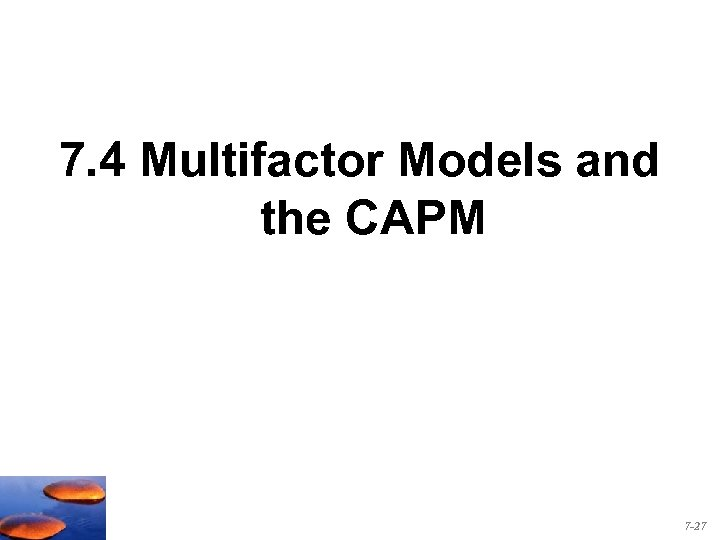7. 4 Multifactor Models and the CAPM 7 -27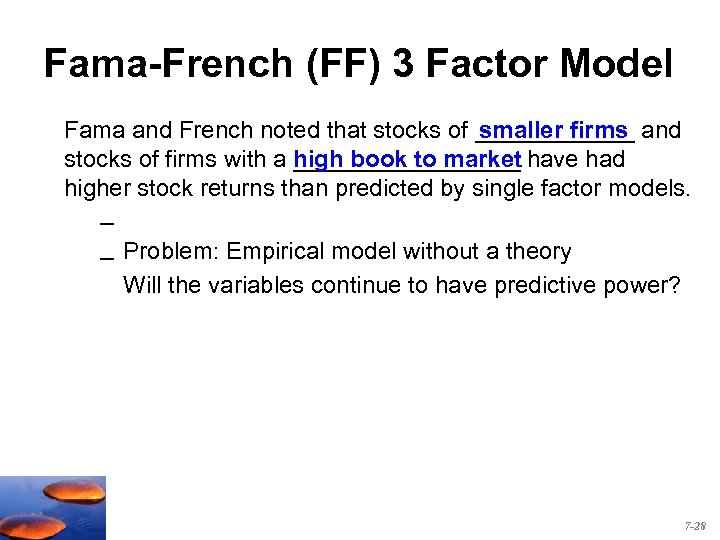Fama-French (FF) 3 Factor Model Fama and French noted that stocks of ______ and smaller firms stocks of firms with a high book to market have had _________ higher stock returns than predicted by single factor models. – – Problem: Empirical model without a theory Will the variables continue to have predictive power? 7 -28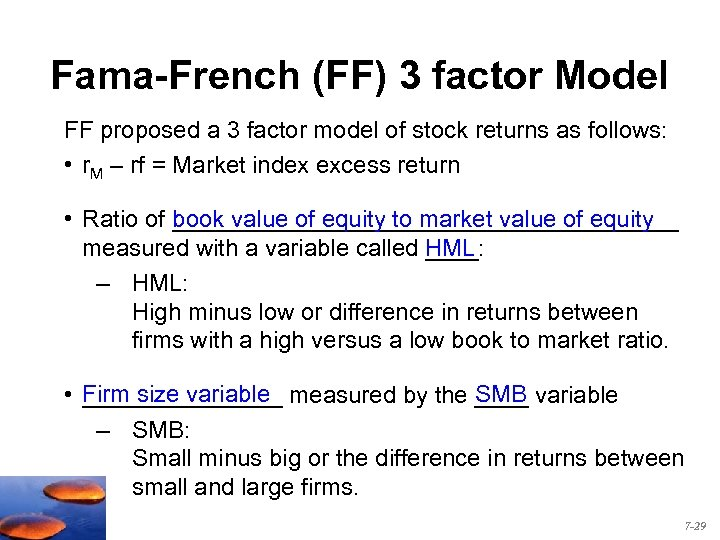Fama-French (FF) 3 factor Model FF proposed a 3 factor model of stock returns as follows: • r. M – rf = Market index excess return • Ratio of ___________________ book value of equity to market value of equity measured with a variable called HML ____: – HML: High minus low or difference in returns between firms with a high versus a low book to market ratio. SMB • Firm size variable measured by the ____ variable ________ – SMB: Small minus big or the difference in returns between small and large firms. 7 -29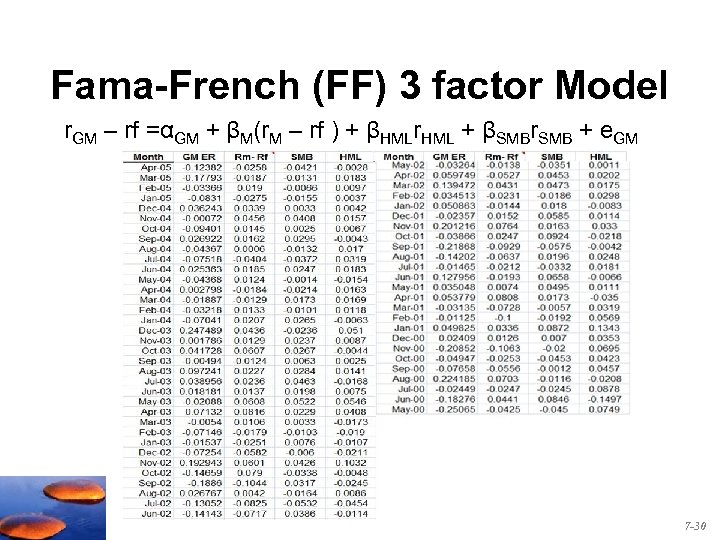Fama-French (FF) 3 factor Model r. GM – rf =αGM + βM(r. M – rf ) + βHMLr. HML + βSMBr. SMB + e. GM 7 -30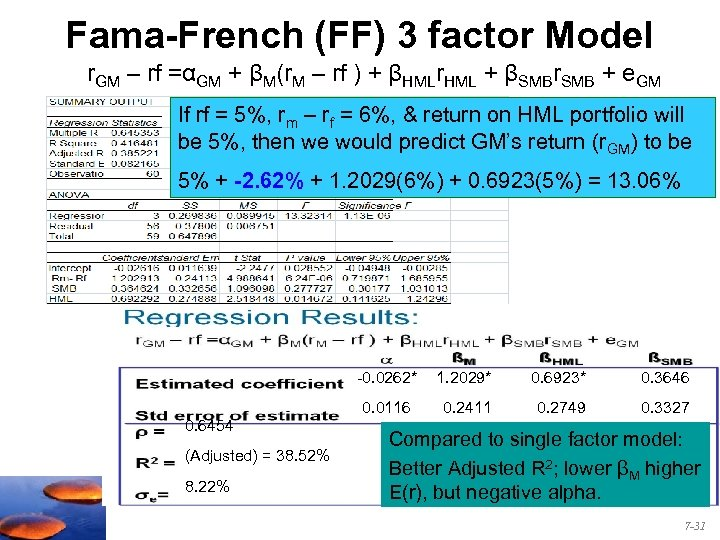Fama-French (FF) 3 factor Model r. GM – rf =αGM + βM(r. M – rf ) + βHMLr. HML + βSMBr. SMB + e. GM If rf = 5%, rm – rf = 6%, & return on HML portfolio will be 5%, then we would predict GM’s return (r. GM) to be 5% + -2. 62% + 1. 2029(6%) + 0. 6923(5%) = 13. 06% -0. 0262* (Adjusted) = 38. 52% 8. 22% 0. 6923* 0. 3646 0. 0116 0. 6454 1. 2029* 0. 2411 0. 2749 0. 3327 Compared to single factor model: Better Adjusted R 2; lower βM higher E(r), but negative alpha. 7 -31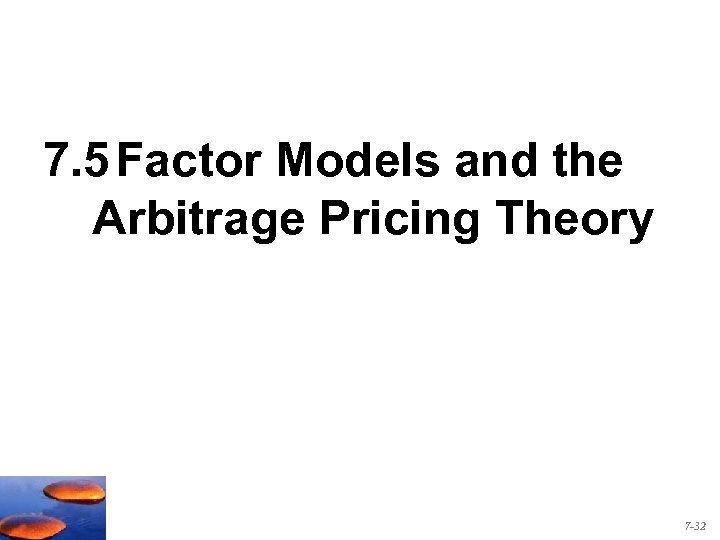7. 5 Factor Models and the Arbitrage Pricing Theory 7 -32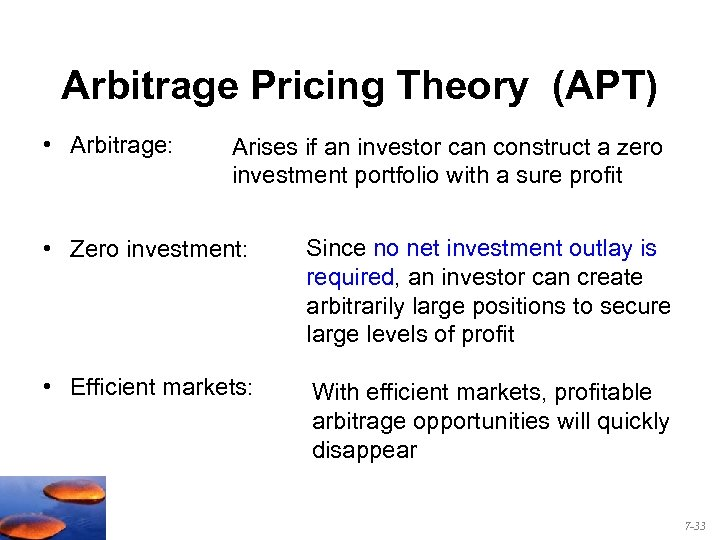Arbitrage Pricing Theory (APT) • Arbitrage: Arises if an investor can construct a zero investment portfolio with a sure profit • Zero investment: Since no net investment outlay is required, an investor can create arbitrarily large positions to secure large levels of profit • Efficient markets: With efficient markets, profitable arbitrage opportunities will quickly disappear 7 -33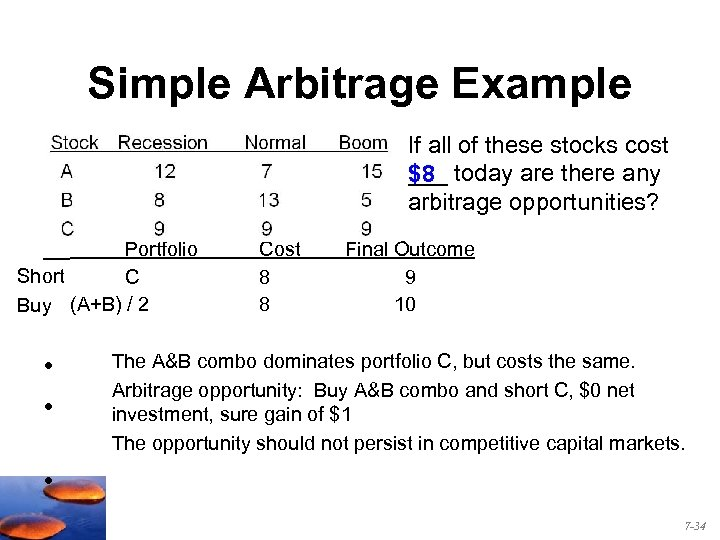Simple Arbitrage Example If all of these stocks cost ___ today are there any \$8 arbitrage opportunities? Portfolio Short C Buy (A+B) / 2 • • Cost 8 8 Final Outcome 9 10 The A&B combo dominates portfolio C, but costs the same. Arbitrage opportunity: Buy A&B combo and short C, \$0 net investment, sure gain of \$1 The opportunity should not persist in competitive capital markets. • 7 -34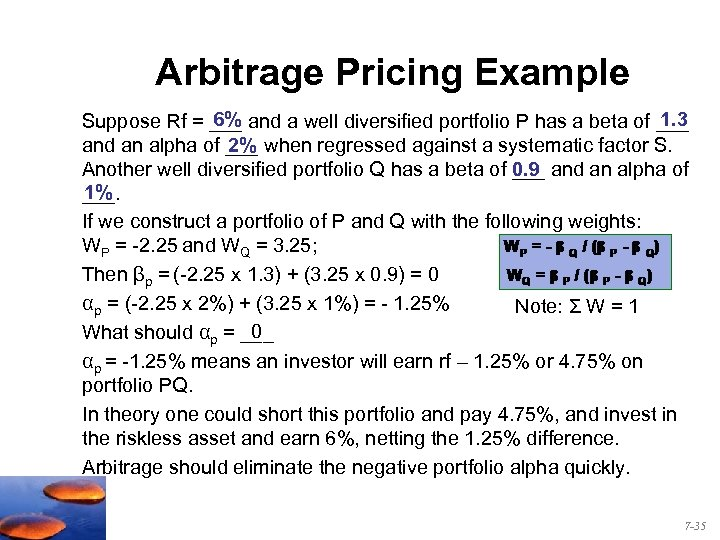Arbitrage Pricing Example 1. 3 6% Suppose Rf = ___ and a well diversified portfolio P has a beta of ___ and an alpha of ___ when regressed against a systematic factor S. 2% Another well diversified portfolio Q has a beta of ___ and an alpha of 0. 9 1% ___. If we construct a portfolio of P and Q with the following weights: WP = -2. 25 and WQ = 3. 25; Then βp = (-2. 25 x 1. 3) + (3. 25 x 0. 9) = 0 αp = (-2. 25 x 2%) + (3. 25 x 1%) = - 1. 25% Note: Σ W = 1 0 What should αp = ___ αp = -1. 25% means an investor will earn rf – 1. 25% or 4. 75% on portfolio PQ. In theory one could short this portfolio and pay 4. 75%, and invest in the riskless asset and earn 6%, netting the 1. 25% difference. Arbitrage should eliminate the negative portfolio alpha quickly. 7 -35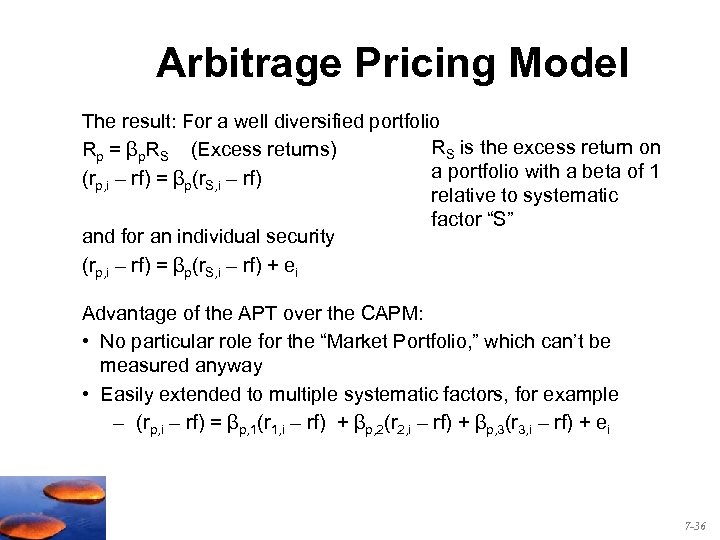Arbitrage Pricing Model The result: For a well diversified portfolio RS is the excess return on Rp = βp. RS (Excess returns) a portfolio with a beta of 1 (rp, i – rf) = βp(r. S, i – rf) relative to systematic factor “S” and for an individual security (rp, i – rf) = βp(r. S, i – rf) + ei Advantage of the APT over the CAPM: • No particular role for the “Market Portfolio, ” which can’t be measured anyway • Easily extended to multiple systematic factors, for example – (rp, i – rf) = βp, 1(r 1, i – rf) + βp, 2(r 2, i – rf) + βp, 3(r 3, i – rf) + ei 7 -36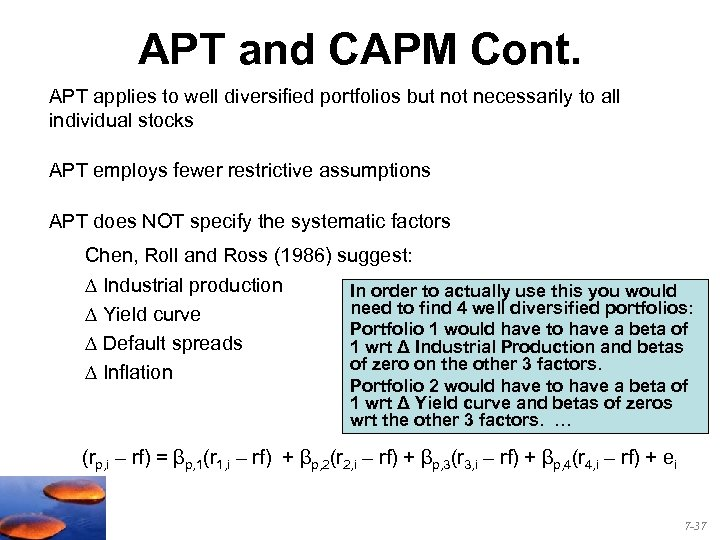APT and CAPM Cont. APT applies to well diversified portfolios but not necessarily to all individual stocks APT employs fewer restrictive assumptions APT does NOT specify the systematic factors Chen, Roll and Ross (1986) suggest: Industrial production Yield curve Default spreads Inflation In order to actually use this you would need to find 4 well diversified portfolios: Portfolio 1 would have to have a beta of 1 wrt Δ Industrial Production and betas of zero on the other 3 factors. Portfolio 2 would have to have a beta of 1 wrt Δ Yield curve and betas of zeros wrt the other 3 factors. … (rp, i – rf) = βp, 1(r 1, i – rf) + βp, 2(r 2, i – rf) + βp, 3(r 3, i – rf) + βp, 4(r 4, i – rf) + ei 7 -37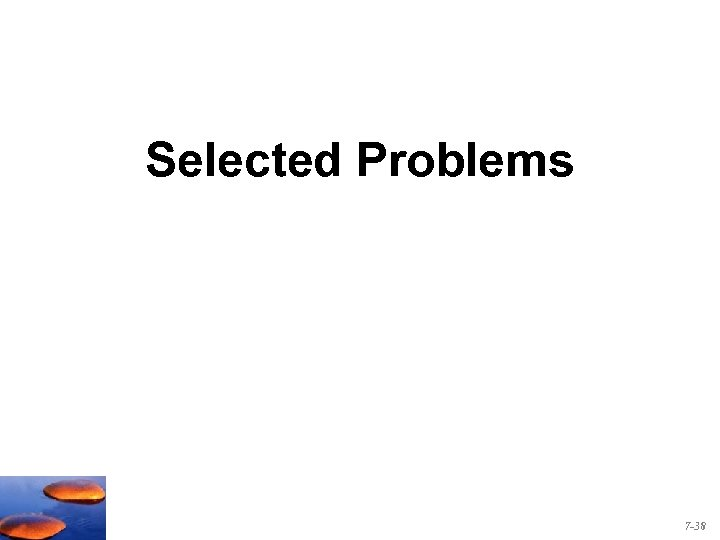Selected Problems 7 -38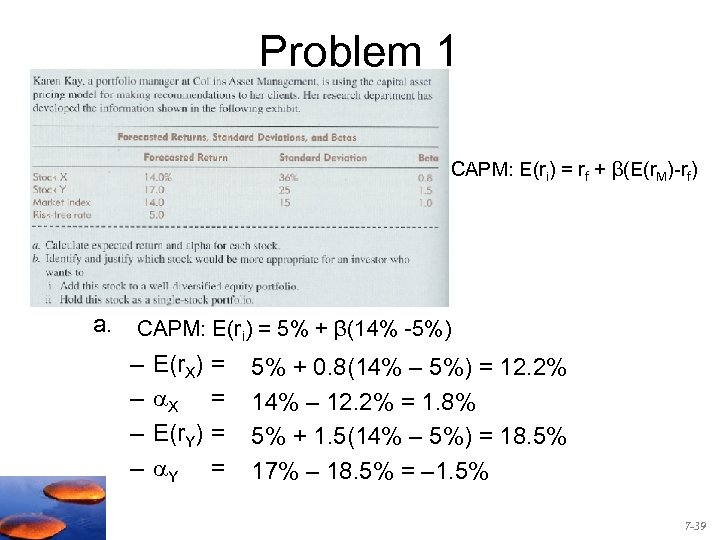Problem 1 CAPM: E(ri) = rf + β(E(r. M)-rf) a. CAPM: E(ri) = 5% + β(14% -5%) – – E(r. X) X E(r. Y) Y = = 5% + 0. 8(14% – 5%) = 12. 2% 14% – 12. 2% = 1. 8% 5% + 1. 5(14% – 5%) = 18. 5% 17% – 18. 5% = – 1. 5% 7 -39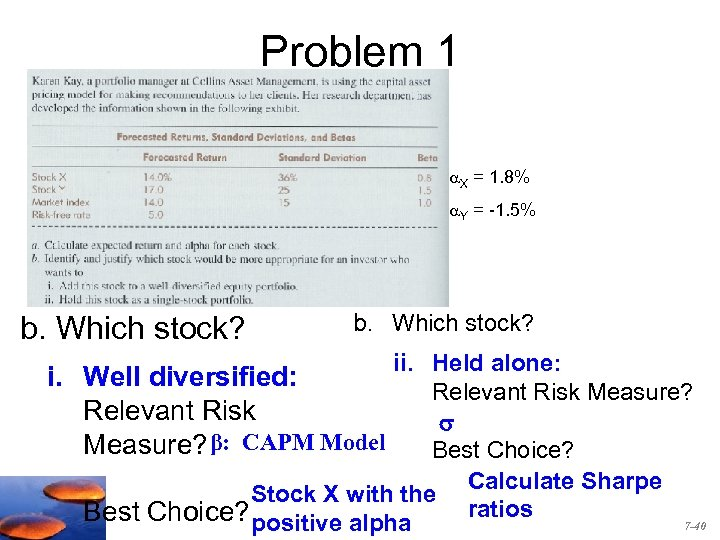Problem 1 X = 1. 8% Y = -1. 5% b. Which stock? ii. Held alone: i. Well diversified: Relevant Risk Measure? Relevant Risk Measure? β: CAPM Model Best Choice? Calculate Sharpe Stock X with the ratios Best Choice? positive alpha 7 -40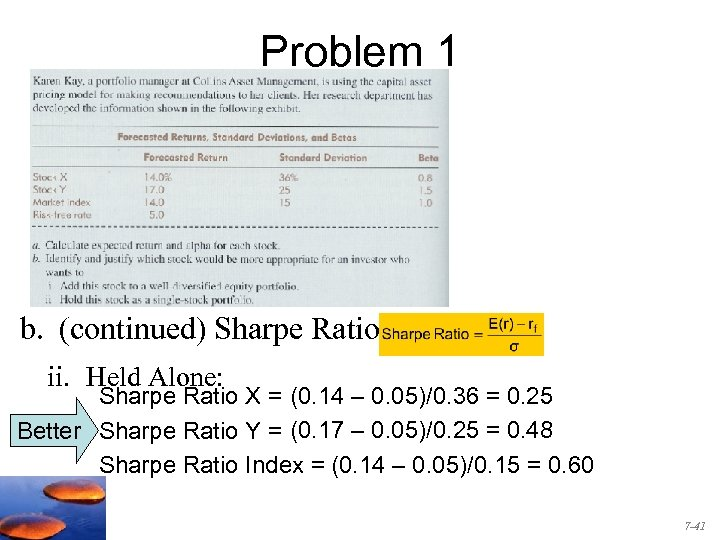Problem 1 b. (continued) Sharpe Ratios ii. Held Alone: Sharpe Ratio X = (0. 14 – 0. 05)/0. 36 = 0. 25 Better Sharpe Ratio Y = (0. 17 – 0. 05)/0. 25 = 0. 48 Sharpe Ratio Index = (0. 14 – 0. 05)/0. 15 = 0. 60 7 -41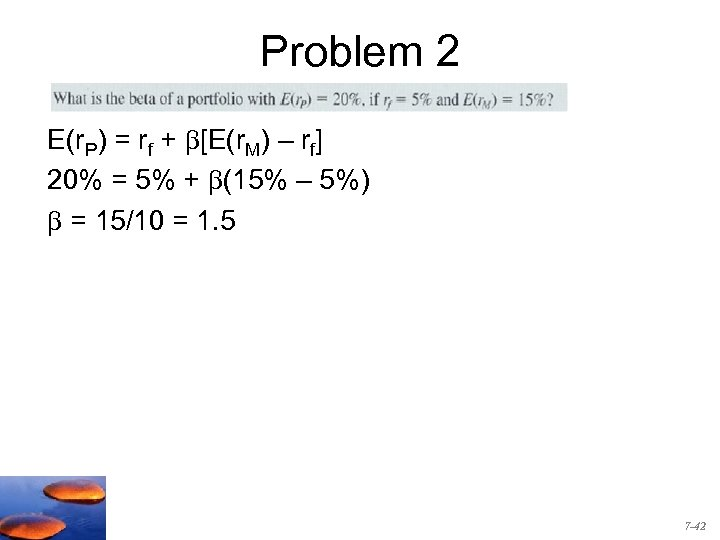Problem 2 E(r. P) = rf + [E(r. M) – rf] 20% = 5% + (15% – 5%) = 15/10 = 1. 5 7 -42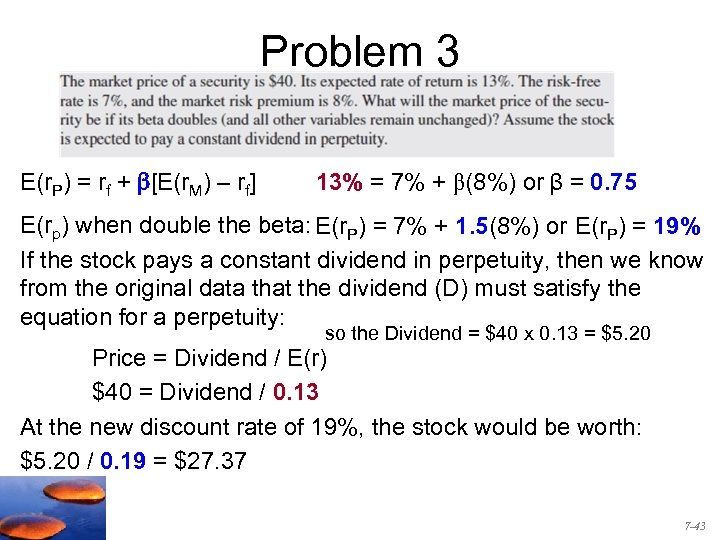Problem 3 E(r. P) = rf + b[E(r. M) – rf] 13% = 7% + β(8%) or β = 0. 75 E(rp) when double the beta: E(r. P) = 7% + 1. 5(8%) or E(r. P) = 19% If the stock pays a constant dividend in perpetuity, then we know from the original data that the dividend (D) must satisfy the equation for a perpetuity: so the Dividend = \$40 x 0. 13 = \$5. 20 Price = Dividend / E(r) \$40 = Dividend / 0. 13 At the new discount rate of 19%, the stock would be worth: \$5. 20 / 0. 19 = \$27. 37 7 -43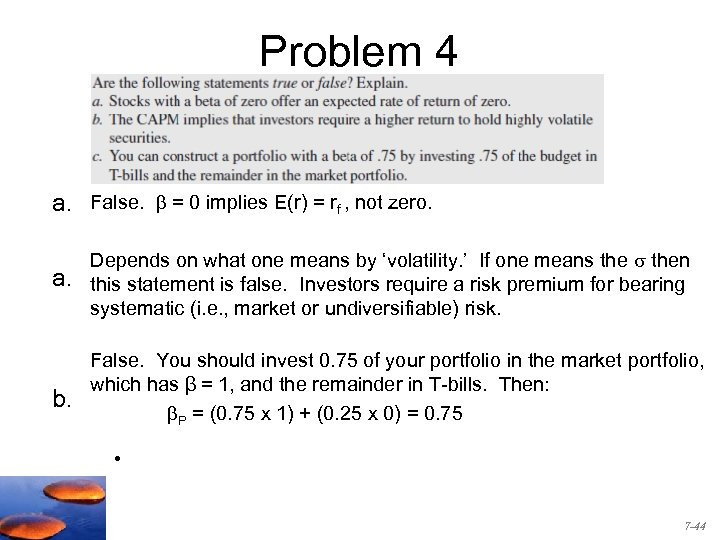Problem 4 a. False. = 0 implies E(r) = rf , not zero. a. Depends on what one means by ‘volatility. ’ If one means then this statement is false. Investors require a risk premium for bearing systematic (i. e. , market or undiversifiable) risk. b. False. You should invest 0. 75 of your portfolio in the market portfolio, which has β = 1, and the remainder in T-bills. Then: P = (0. 75 x 1) + (0. 25 x 0) = 0. 75 • 7 -44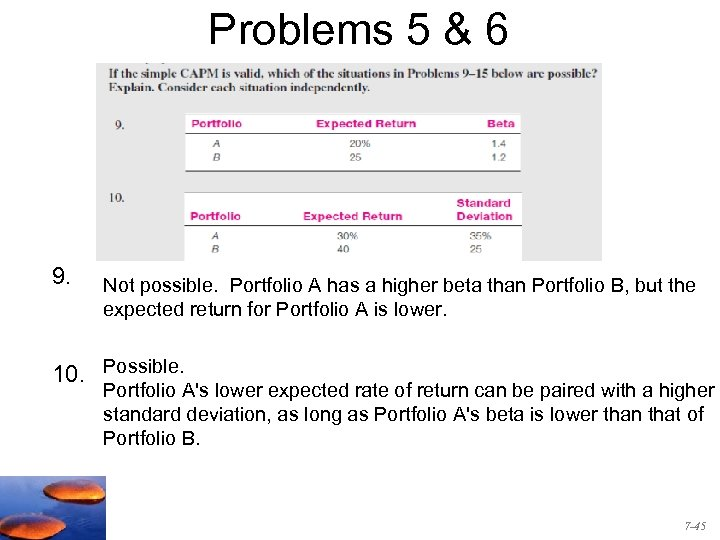Problems 5 & 6 9. Not possible. Portfolio A has a higher beta than Portfolio B, but the expected return for Portfolio A is lower. 10. Possible. Portfolio A's lower expected rate of return can be paired with a higher standard deviation, as long as Portfolio A's beta is lower than that of Portfolio B. 7 -45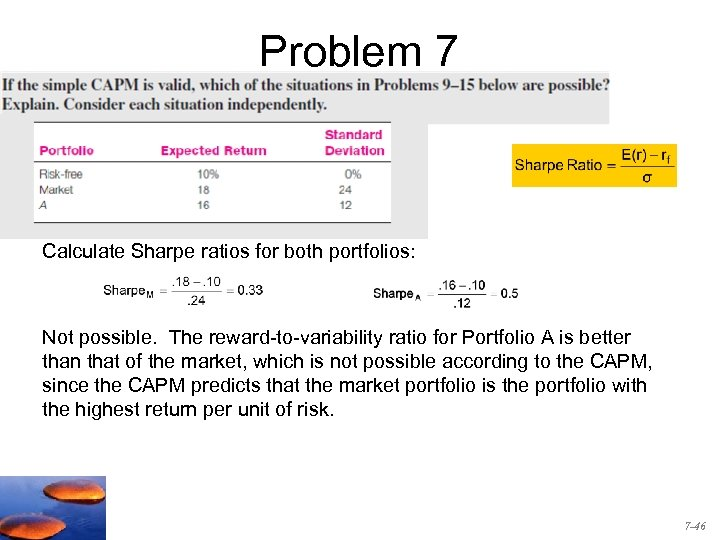Problem 7 Calculate Sharpe ratios for both portfolios: Not possible. The reward-to-variability ratio for Portfolio A is better than that of the market, which is not possible according to the CAPM, since the CAPM predicts that the market portfolio is the portfolio with the highest return per unit of risk. 7 -46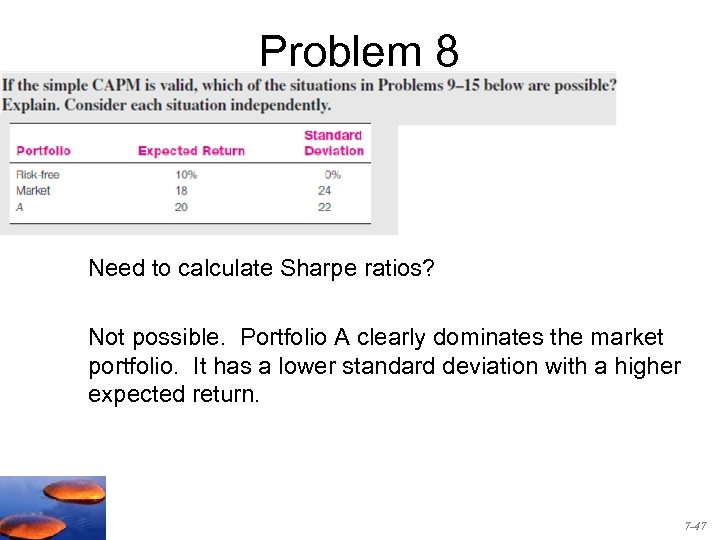Problem 8 8. Need to calculate Sharpe ratios? Not possible. Portfolio A clearly dominates the market portfolio. It has a lower standard deviation with a higher expected return. 7 -47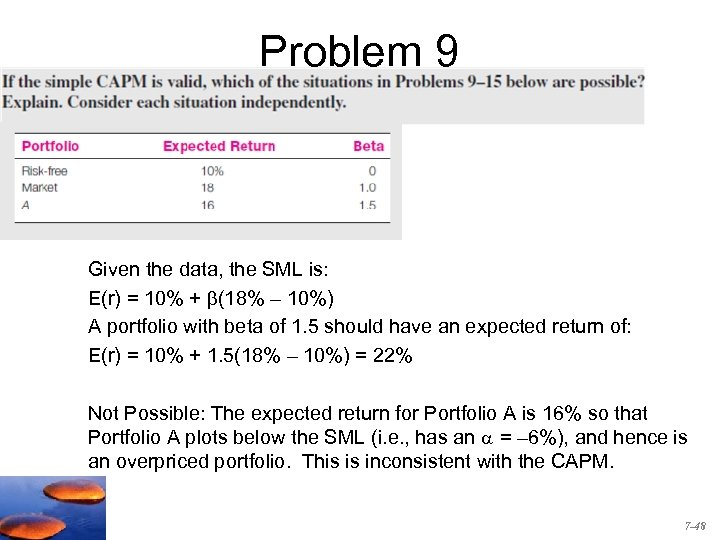Problem 9 9. Given the data, the SML is: E(r) = 10% + (18% – 10%) A portfolio with beta of 1. 5 should have an expected return of: E(r) = 10% + 1. 5(18% – 10%) = 22% Not Possible: The expected return for Portfolio A is 16% so that Portfolio A plots below the SML (i. e. , has an = – 6%), and hence is an overpriced portfolio. This is inconsistent with the CAPM. 7 -48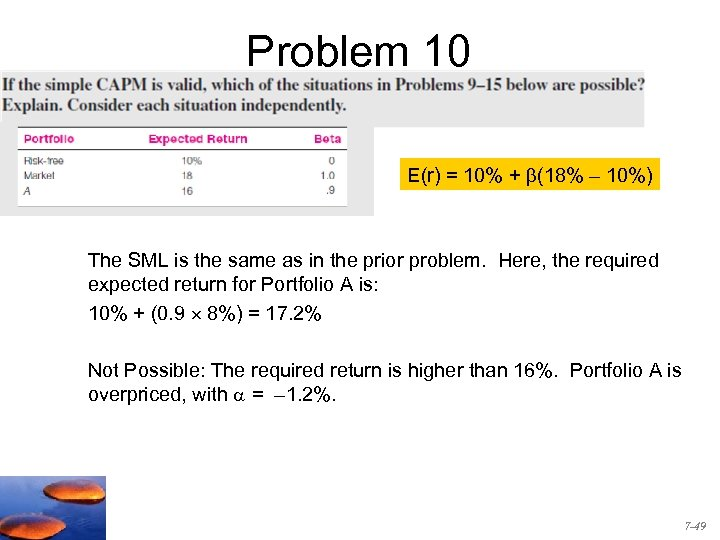Problem 10 E(r) = 10% + (18% – 10%) The SML is the same as in the prior problem. Here, the required expected return for Portfolio A is: 10% + (0. 9 8%) = 17. 2% Not Possible: The required return is higher than 16%. Portfolio A is overpriced, with = – 1. 2%. 7 -49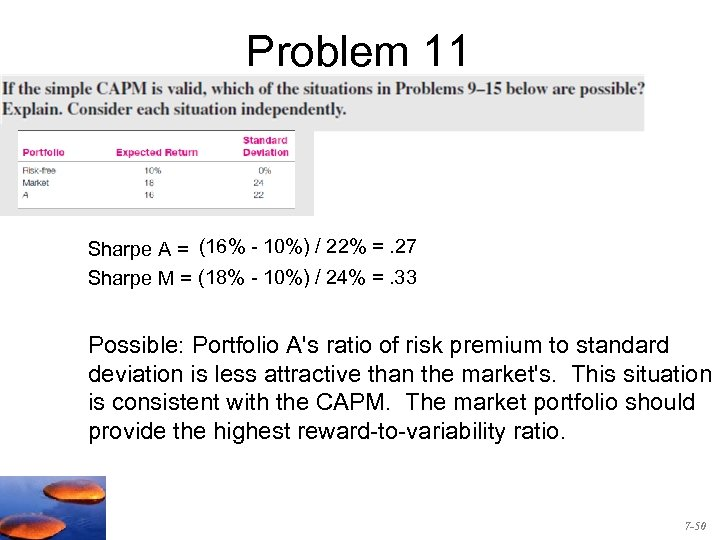Problem 11 Sharpe A = (16% - 10%) / 22% =. 27 Sharpe M = (18% - 10%) / 24% =. 33 Possible: Portfolio A's ratio of risk premium to standard deviation is less attractive than the market's. This situation is consistent with the CAPM. The market portfolio should provide the highest reward-to-variability ratio. 7 -50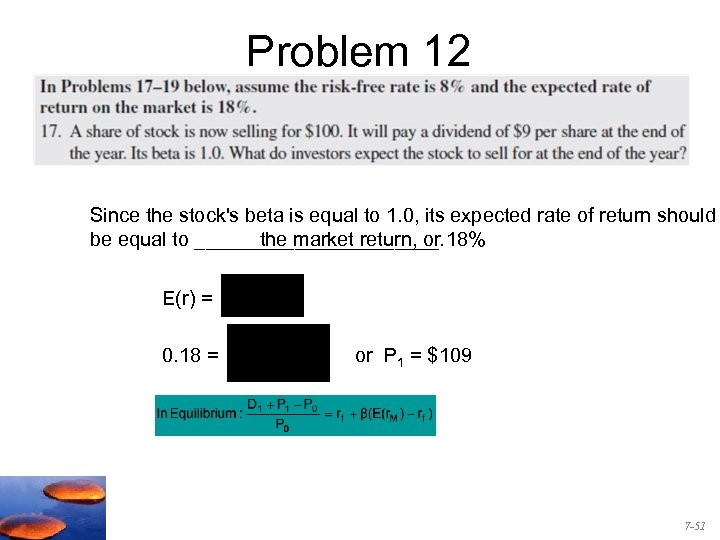Problem 12 Since the stock's beta is equal to 1. 0, its expected rate of return should the market return, or be equal to ___________. 18% E(r) = 0. 18 = or P 1 = \$109 7 -51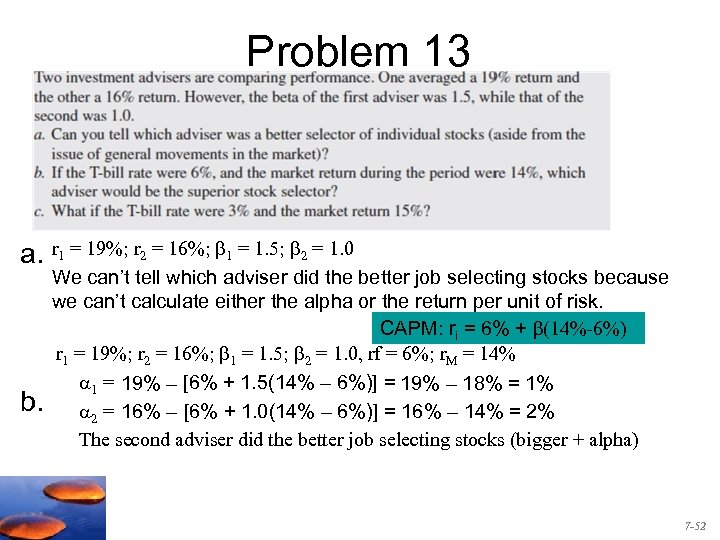Problem 13 a. r 1 = 19%; r 2 = 16%; 1 = 1. 5; 2 = 1. 0 b. We can’t tell which adviser did the better job selecting stocks because we can’t calculate either the alpha or the return per unit of risk. CAPM: ri = 6% + β(14%-6%) r 1 = 19%; r 2 = 16%; 1 = 1. 5; 2 = 1. 0, rf = 6%; r. M = 14% 1 = 19% – [6% + 1. 5(14% – 6%)] = 19% – 18% = 1% 2 = 16% – [6% + 1. 0(14% – 6%)] = 16% – 14% = 2% The second adviser did the better job selecting stocks (bigger + alpha) 7 -52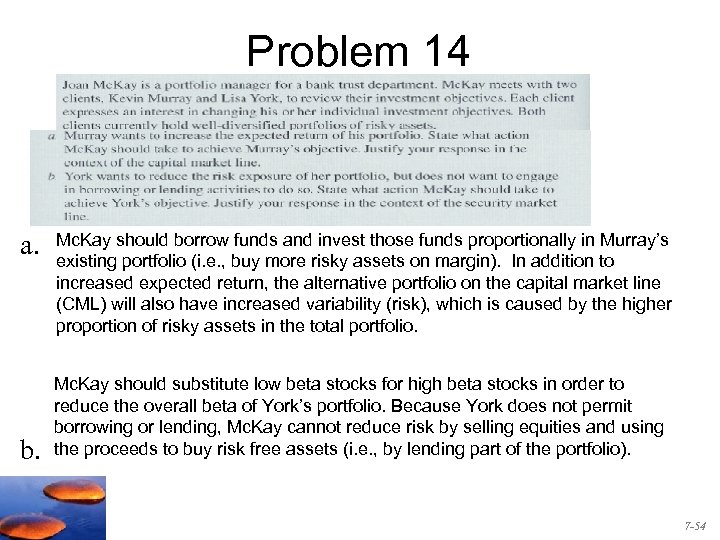Problem 14 a. b. Mc. Kay should borrow funds and invest those funds proportionally in Murray’s existing portfolio (i. e. , buy more risky assets on margin). In addition to increased expected return, the alternative portfolio on the capital market line (CML) will also have increased variability (risk), which is caused by the higher proportion of risky assets in the total portfolio. Mc. Kay should substitute low beta stocks for high beta stocks in order to reduce the overall beta of York’s portfolio. Because York does not permit borrowing or lending, Mc. Kay cannot reduce risk by selling equities and using the proceeds to buy risk free assets (i. e. , by lending part of the portfolio). 7 -54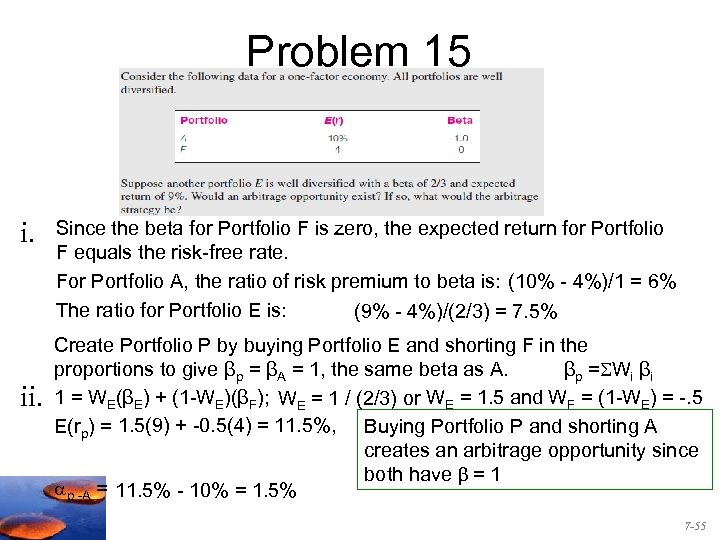Problem 15 i. ii. Since the beta for Portfolio F is zero, the expected return for Portfolio F equals the risk-free rate. For Portfolio A, the ratio of risk premium to beta is: (10% - 4%)/1 = 6% The ratio for Portfolio E is: (9% - 4%)/(2/3) = 7. 5% Create Portfolio P by buying Portfolio E and shorting F in the proportions to give βp = βA = 1, the same beta as A. βp = Wi βi 1 = WE(βE) + (1 -WE)(βF); WE = 1 / (2/3) or WE = 1. 5 and WF = (1 -WE) = -. 5 E(rp) = 1. 5(9) + -0. 5(4) = 11. 5%, Buying Portfolio P and shorting A creates an arbitrage opportunity since both have β = 1 p, -A = 11. 5% - 10% = 1. 5% 7 -55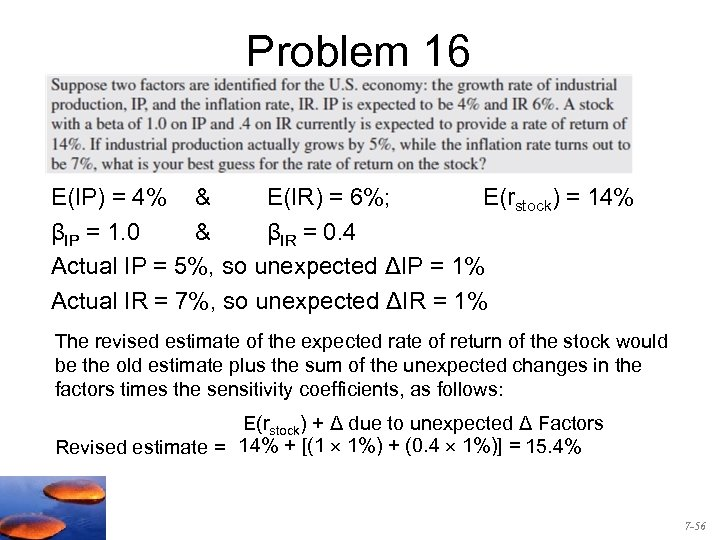Problem 16 E(IP) = 4% & E(IR) = 6%; E(rstock) = 14% βIP = 1. 0 & βIR = 0. 4 Actual IP = 5%, so unexpected ΔIP = 1% Actual IR = 7%, so unexpected ΔIR = 1% The revised estimate of the expected rate of return of the stock would be the old estimate plus the sum of the unexpected changes in the factors times the sensitivity coefficients, as follows: E(rstock) + Δ due to unexpected Δ Factors Revised estimate = 14% + [(1 1%) + (0. 4 1%)] = 15. 4% 7 -56